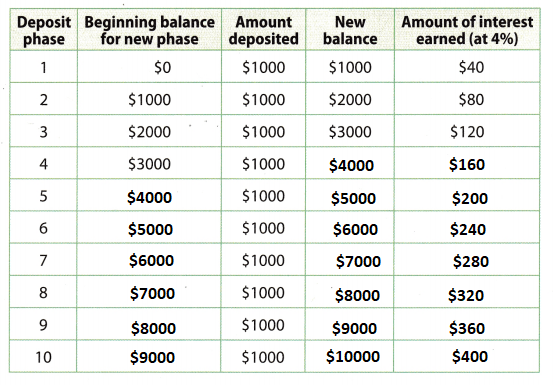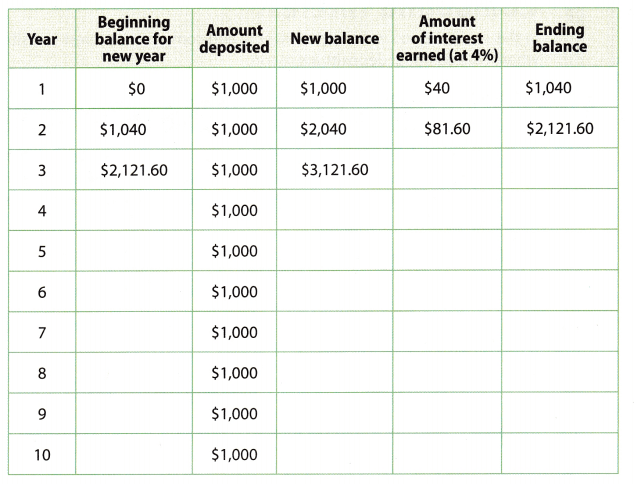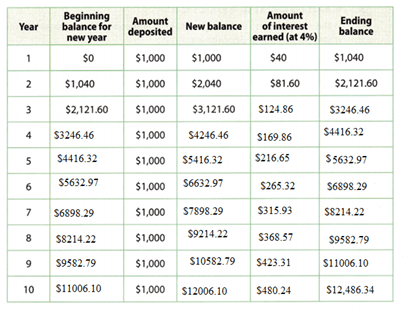# Texas Go Math Grade 8 Lesson 16.2 Answer Key Saving and Investing

Refer to our Texas Go Math Grade 8 Answer Key Pdf to score good marks in the exams. Test yourself by practicing the problems from Texas Go Math Grade 8 Lesson 16.2 Answer Key Saving and Investing.

## Texas Go Math Grade 8 Lesson 16.2 Answer Key Saving and Investing

Explore Activity 1

Calculating Simple Interest

Interest is money paid by banks and others for the use of depositors’ money. Simple interest is earned using the formula l = Prt, where l is the amount of interest, P is the principal, or the original amount deposited, r is the interest rate expressed as a decimal, and f is the time in years. Simple interest is paid at the end of the term based only on the principal at the beginning.

Adan makes regular deposits to a savings account to save money for college. He deposits $1000 at the start of each year into an account that pays 4% simple interest at the end of each year. He does not deposit the interest. A. How much interest does Adan’s account earn the first year? l = Prt Use the formula for simple interest. l = 1000 × __________ × 1 = __________ Substitute and simplify Adan’s account earns ___________ the first year. Answer: Given, Adan makes regular deposits to a savings account to save money for college. He deposits$1000 at the start of each year into an account that pays 4% simple interest at the end of each year.
He does not deposit the interest.
I = Prt
P = 1000
r = 0.04
t = 1
I = 1000 × 0.04 × 1
I = 40
Adan’s account earns $40the first year. B. Complete the table to show how the interest earned grows over time.Answer:Explanation: 4. Beginning balance =$3000
Amount deposited = $1000 3000 + 1000 =$4000
So, new balance = $4000 Amount of interest earned at 4% = 4000 × 0.04 =$160
5. Beginning balance = $4000 Amount deposited =$1000
4000 + 1000 = $5000 So, new balance =$5000
Amount of interest earned at 4% = 5000 × 0.04 = $200 6. Beginning balance =$5000
Amount deposited = $1000 5000 + 1000 =$6000
So, new balance = $6000 Amount of interest earned at 4% = 6000 × 0.04 =$240
7. Beginning balance = $6000 Amount deposited =$1000
6000 + 1000 = $7000 So, new balance =$7000
Amount of interest earned at 4% = 7000 × 0.04 = $280 8. Beginning balance =$7000
Amount deposited = $1000 7000 + 1000 =$8000
So, new balance = $8000 Amount of interest earned at 4% = 8000 × 0.04 =$320
9. Beginning balance = $8000 Amount deposited =$1000
8000 + 1000 = $9000 So, new balance =$9000
Amount of interest earned at 4% = 9000 × 0.04 = $360 10. Beginning balance =$9000
Amount deposited = $1000 9000 + 1000 =$10000
So, new balance = $10000 Amount of interest earned at 4% = 10000 × 0.04 =$400

Reflect

Question 1.
How much interest did Adan’s account earn from the initial deposit to the end of year 5? from the start of year 6 to the end of year 10? How do these values compare? Explain.
Adan deposits $1000 at the start of each year. The income earned at year 10 =$400
The income earned in the next 5 years = $600 Adding$600 to the initial deposit = 600 + 1000 = $1600 More interest is earned in later years as deposits are made. If money is saved regularly, the amount of interest increases over time. Question 2. What was the total amount saved from the initial deposit to the end of year 5? from the start of year 6 to the end of year 10? Include the amount contributed and the interest. Answer: The income earned in the next 5 years =$600
So, new balance = $5000 Adding both to know the total amount saved from the initial deposit to the end of year 5.$5000 + $600 =$5600
Beginning balance = $5000 Amount deposited =$1000
5000 + 1000 = $6000 So, new balance =$6000
$6000 +$600 = $6600 Explore Activity 2 Calculating Compound interest Compound interest is interest paid not only on the principal but also on any interest that has already been earned. Every time interest is calculated, the interest is added to the principal for future interest calculations. The calculation can be made more than once a year, but in this lesson only interest compounded annually will be found. The formula for compound interest is A = P(1 + r)t, where P is the principal, r is the interest rate expressed as a decimal, t is the time in years, and A is the amount in the account after t years if no withdrawals were made. Lilly makes regular deposits to a savings account to save money for retirement. She deposits$1000 each year, and her account earns interest compounded annually at a rate of 4%.
A. How much interest does Lilly earn the first year?
A = P(1 + r)t Use the formula for compound interest.
A = 1000 × (1 + __________) 1 Substitute
A = _________ Simplify
So, Lilly’s account earns __________ – $1000 = __________ the first year. Answer: A = P(1 + r)t Use the formula for compound interest. A = 1000 × (1 + 0.04) 1 A = 1040 So, Lilly’s account earns$1040- $1000 =$40 the first year.

B. Complete the table to show how the amount in the account accumulates over time. Round all values to the nearest cent.Beginning balance for the new year = $0. The amount deposited in account =$1000.
Interest = 4%
The formula for compound interest is A = P(1 + r/100)t
P = principle
R = interest rate.
T = time
A = $1000(1 + 4/100)1 =$1000 (1.04)
Ending balance = $1040 =$1040 – $1000 = 40 Interest for 1 year = 40. As for the 1 year calculation. For the second year the beginning balance is$1040.
The ending balance is $2121.60. The interest for the second year =$81.60.
For the 3rd year the beginning balance is $2121.60. The ending balance is$3246.46.
The interest for the second year = $124.86. For the second year the beginning balance is$1040.
The ending balance is $212160. The interest for the second year =$81.60.
For the second year the beginning balance is $1040. The ending balance is$212160.
The interest for the second year = $81.60. For the second year the beginning balance is$1040.
The ending balance is $212160. For the 10 years the beginning balance, interest and ending balance is in the table. Reflect Question 3. How much interest did Lilly’s account earn from the initial deposit to the end of year 5? from the start of year 6 to the end of year 10? Answer: The interest earned from the initial deposit to the end of 5 years =$40 + $81.60 +$124.86 + $169.86 +$216.65 = $632.97. The interest earned from 6 years to 10 years =$265.32 + $315.93 +$368.57 +$423.31 +$480.24 = $1853.37. Question 4. Compare the interest earned during the two five-year periods. Explain the difference. Answer: The interest for the first 5 years =$632.97.
The interest for after 5 years = $1853.37. The interest after 5 years has increased almost 3 times to the first five years. Because every year a new amount is deposited. So, the interest increases. Question 5. Compare the final balance in this Explore Activity to the total amount deposited and earned in interest in Explore Activity 1 (see Reflect question 2). What can you conclude? Answer: In explore 1 the total was$5600 + $6600 =$12200.
In explore 2 the total final balance is $12486.34. The explore 2 earned more compared to the explore 1. Your Turn Question 6. Marlena saved$50 in an account earning 3.5% simple interest. How much more interest would her account earn in 10 years if her account earned interest compounded annually instead of simple interest?
Given that,
Marlena saved in the account = $50. Simple interest = 3.5%. Number of years = 10. The formula for compound interest is A = P(1 + r)t P = principle R = interest rate. T = time A =$50(1 + 3.5)10
=$50 (1.410) =$70.5
= $70.5 –$50 = 20.5
The compounded interest for 10 years is 20.5

Question 1.
Gina deposits $150 at the start of each year into a college savings account that pays 4% simple interest at the end of each year. She does not deposit the interest she earns each year. How much total interest will Gina earn on her deposits through the end of the fifth year? (Explore Activity 1) Answer: Given that, Gina deposits into a college savings account =$150.
Simple interest = 4%.
Number of years = 5
Formula for simple interest equal to SI = PTR/100
Here,
P = actual money = $150. T = time = 5 years. R = interest rate = 4% Simple interest for 5 years =$150 × 5 × 4/100 = $300. The total interest will Gina earn on her deposits through the end of the fifth year =$300.

Question 2.
Fredo deposits $75 each year in an account earning 3% interest compounded annually. If he deposits an additional$75 per year and does not make any withdrawals, how much interest will the account earn in the fourth year? (Explore Activity 2)
Given that,
Fredo deposits each year in an account = $75 Interest = 3%. Interest for one year The formula for compound interest is A = P(1 + r/100)t A =$75(1 + 3/100)¹
A = $75(1.03) A =$9.75
For second year deposit = $75 +$75 = 150.
Interest for 2 years = 9.75 + 9.75 = 19.5
The interest for the account earn in the fourth year = $97.5 Question 3. Huan deposited$850 into a college savings account earning 4.8% interest compounded annually. He also deposited $850 into a second account earning 4.8% simple interest. He made no additional deposits. (Example 1) a. How much interest does the first account earn in 10 years? Answer: Huan deposited into a college savings account =$ 850.
Earning an interest = 4.8%.
Number of years = 10
The formula for compound interest is A = P(1 + r/100)t
A = $850(1 + 4.8/100)10 A =$850(1.5981)
A = $1358.3 The compound interest for 10 years =$1358.3 – $850 =$508.3

b. How much interest does the second account earn in 10 years?
Huan deposited into a college savings account = $850. Earning an interest = 4.8%. Number of years = 10 Formula for simple interest equal to SI = PTR/100 Here, P = actual money =$850.
T = time = 10 years.
R = interest rate = 4.8%
Simple interest for 10 years = $850 × 10 × 4.8/100 =$408

c. After 10 years, which account earned more interest? How much more?
The compound interest for 10 years = $508.3 The simple interest for 10 years =$408.
$508.3 –$408 = $100.3 Compound interest has more interest than simple interest.$100.3 is more than simple interest.

Question 4.
Andreas invested $1000 in a savings account. After 4 years, the account had earned a total of$112 simple interest without any additional deposits. What was his interest rate?
Given,
Andreas invested $1000 in a savings account. After 4 years, the account had earned a total of$112 in simple interest without any additional deposits.
I = Prt
112 = 1000 × r × 4
112 = 4000 × r
r = 0.028
rate of interest = 2.8%

Question 5.
Hei has $1500 in a retirement account earning 5% interest compounded annually. Each year after the first, she makes additional deposits of$1500. After 5 years, what was her account balance if she did not make any withdrawals?
Given,
Hei has $1500 in a retirement account earning 5% interest compounded annually. Each year after the first, she makes additional deposits of$1500.
After 1 year:
1500 × (1 + 0.05) = 1500 × 1.05 = $1575 After 2 years: Adding the interest from the previous year to this year. 1575 + 1500 = 3075 3075 × (1 + 0.05) = 3075 × 1.05 =$3228.75
After 3 years:
Adding the interest from the previous year to this year.
3228.75 + 1500 = 4728.75
4728.75× (1 + 0.05) = 4728.75× 1.05 = $4965.18 After 4 years: Adding the interest from the previous year to this year. 4965.18 + 1500 = 6465.18 6465.18 × (1 + 0.05) = 6465.18 × 1.05 =$6788.44
After 5 years:
Adding the interest from the previous year to this year.
6788.44 + 1500 = 8288.44
8288.44 × (1 + 0.05) = 8288.44 × 1.05 = $8702.87 Question 6. Lester deposited$400 into a savings account earning 4.5% simple interest, and $450 into an investment account earning 3.2% interest compounded annually. What was the total interest he earned in 3 years? Justify your reasoning. Answer: Given, Lester deposited$400 into a savings account earning 4.5% simple interest, and $450 into an investment account earning 3.2% interest compounded annually. Formula for simple interest is I = prt I = 400 × 0.045 × 3 = 54 Compound balance after 3 years A = p(1 + r)t A = 450 × (1 + 0.032)³ A = 494.597 Compund interest earned is 494.59 – 450 = 44.59 Total = 54 + 44.59 = 98.59 Question 7. Randee invested$1000 for college in an account earning 5% simple interest. When she withdrew the investment, she had earned a total of $550 in interest. How long was the money invested? Justify your reasoning. Answer: Given, Randee invested$1000 for college in an account earning 5% simple interest.
When she withdrew the investment, she had earned a total of $550 in interest. The formula for simple interest A = P(1 + rt) 1550 = 1000 (1 + (0.05 × t)) 1.55 = 1 + 0.05 × t 1.55 – 1 = 0.05 × t 0.55 = 0.05t t = 11 Question 8. Critical Thinking Is it possible for an amount of money invested in an account earning simple interest to earn more interest than the same amount of money invested at the same rate in an account earning interest compounded annually? Explain. Answer: Comparing the simple interest and compounded interest. The compounded interest is more beneficial. In this the funds increase fastly. Because the interest is calculated on the accumulated interest over the time. H.O.T. Focus on Higher Order Thinking Question 9. Multiple Representations The graph shows how the values of two accounts increase over time. The line represents$50 invested in an account paying 5% simple interest, and the curve represents $50 invested in an account paying 5% interest compounded annually. Write an equation for the line and for the curve. Assume no additional deposits were made to either account.Answer: The line represents$50 invested in an account paying 5% simple interest.
The curve represents $50 invested in an account paying 5% interest compounded annually The equation for the line is y = 5x + 50. The equation for the curve = dy/dx = 5x + 50. Question 10. Critique Reasoning Marco says he will earn more interest on his$100 savings if he gets 4% interest compounded annually than if he gets 5% simple interest. How many years does he have to keep the money in the bank without withdrawing any to be right? Justify your reasoning.
Given,
Marco says he will earn more interest on his $100 savings if he gets 4% interest compounded annually than if he gets 5% simple interest. If he gets 5% simple interest on$100 = 5/100 × 100 = $5 Thus the compound interest must be greater than$5.
Let us calculate the compound interest over the years.
Year 1: 4/100 × 100 = $4 Year 2: 100 +$4 = $104 = 4/100 × 104 =$4.16
Year 3: Interest from the 2nd year will be added this year.
104 + 4.16 = 108.16
4/100 × 108.16 = $4.33 Year 4: Interest from the 3rd year will be added this year. 108.16 + 4.33 =$112.49
4/100 × 112.49 = $4.49 Year 5: Interest from the 4th year will be added this year. 112.49 + 4.49 = 116.99 4/100 × 116.99 =$4.68
Year 6: Interest from the 5th year will be added this year.
116.99 + 4.68 = 121.67
4/100 × 121.67= $4.87 Year 7: Interest from the 6th year will be added this year. 121.67 + 4.87 = 126.54 4/100 × 126.54=$5.06
Hence in the 7th year, Marco will get the compound interest greater than $5, so he will have to keep money in the bank without withdrawing for 6 years. Question 11. Critique Reasoning Parker invested$6,500 for 2 years, part at 6% interest compounded annually and part at 5% simple interest. He earned three times as much interest in the account paying compound interest as in the account paying simple interest. Can Parker model this situation using the equation x(1 + 0.06)2 = 3(6500 – x)(0.05)(2), where x is the initial amount in the 6% account and 6500 – x is the amount in the 5% account? Explain.
Parker invested for 2 years = $6500 Interest = 6% The formula for compound interest is A = P(1 + r/100)t A =$6500(1 + 6/100)2
A = $6500(1.123) A =$6500(1.123)
A = $7299.5 The compounded interest for 2 years =$7299.5 – $6500 =$799.5.
P = actual money = $6500. T = time = 2 years. R = interest rate = 5% Simple interest for 10 years =$6500 × 2 × 5/100 = $650 The equation x(1 + 0.06)² = 3(6500 – x)(0.05)(2). where, x is the initial amount in the 6% account and 6500 – x is the amount in the 5% account. Initial amount =$6500
$6500(1 + 0.06)² = 3(6500 –$6500)(0.05)(2).
= $7303.4 =$0